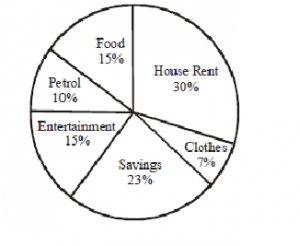#IBPS Clerk Main: Quants Day 18

Hello Aspirants,

Welcome to Online Quant Section in AffairsCloud.com. We are starting IBPS Clerk course 2015 and we are creating sample questions in Quantitative Aptitude section, type of which will be asked in IBPS Clerk Main Exam.

Stratus – IBPS Clerk Course 2015[flipclock]

1. 5x2 + 17x – 12 = 0, 5y2 – 18y + 9 = 0
A) x > y
B) x < y
C) x ≥ y
D) x ≤ y
E) x = y or relationship cannot be determined
D) x ≤ y
Explanation:

5x2 + 17x – 12 = 0
5x2 + 20x – 3x – 12 = 0
Gives x = -4, 3/5
5y2 – 18y + 9 = 0
5y2 – 15y – 3y + 9 = 0
Gives y = 3/5, 3
Put on number line
-4          3/5               3

2. 3x2 + 14x + 8 = 0, 3y2 – 4y – 4 = 0
A) x > y
B) x < y
C) x ≥ y
D) x ≤ y
E) x = y or relationship cannot be determined
D) x ≤ y
Explanation:

3x2 + 14x + 8 = 0
3x2 + 12x + 2x + 8 = 0
Gives x = -4, -2/3
3y2 – 4y – 4 = 0
3y2 – 6y + 2y – 4 = 0
Gives y = -2/3, 2
Put on number line
-4       -2/3         2

3. x = √1849, y2 = 1156
A) x > y
B) x < y
C) x ≥ y
D) x ≤ y
E) x = y or relationship cannot be determined
A) x > y
Explanation:

x = √1849
Gives, x = 43
y2 = 1156
Gives y = -34, 34
Put on number line
-34          34              43

4. 4x + 5y = 75, 5x + 4y = 69
A) x > y
B) x < y
C) x ≥ y
D) x ≤ y
E) x = y or relationship cannot be determined
B) x < y
Explanation:

Multiply first equation by 4 and second by 5 and subtract
16x + 20y = 300, 25x + 20y = 345
Subtract
-9x = -45
Gives x = 5
Put x = 5 in one of the given equations, and find y
4(5) + 5y = 75
Gives y = 11
Put on number line
5      11

5. 6x2 – 5x – 6 = 0, 3y2 – 7y – 6 = 0
A) x > y
B) x < y
C) x ≥ y
D) x ≤ y
E) x = y or relationship cannot be determined
E) x = y or relationship cannot be determined
Explanation:

6x2 – 5x – 6 = 0
6x2 – 9x + 4x – 6 = 0
Gives, x = -2/3, 3/2
3y2 – 7y – 6 = 0
3y2 – 9y + 2y – 6 = 0
Gives, y = -2/3, 3
Put on number line
-2/3           3/2            3
When x = -2/3, x ≤ y
When x = 3/2, x > y(-2/3) and also x < y(3)
No so relationship.

DIRECTIONS (6 – 10) : These questions are to be answered on the basis of the pie chart given below showing how a person’s monthly salary is distributed over different expense heads.1. For a person, whose monthly salary is Rs 6,000, how many items are there on which he has to spend more than Rs 1,000 per month ?
A) None
B) 1
C) 2
D) 3
E) 4
C) 2
Explanation:

Shortcut-
(x/100)*6000 = 1000, gives x = 16.67%
So the ones having more expense % than 16.67% which is house rent and savings

2. The annual saving for such a person will be approximately:
A) Rs 15050
B) Rs 16560
C) Rs 15500
D) Rs 16500
E) None of these
B) Rs 16560
Explanation:

Savings over month = (23/100)*6000 = 1380
So annually = 1380*12 = 16560

3. The monthly salary for a person who follows the same expense pattern, but has a petrol expense of Rs 500 per month is
A) Rs 2500
B) Rs 3500
C) Rs 5000
D) Rs 6500
E) Rs 6600
C) Rs 5000
Explanation:

(10/100)*x = 500

4. If the monthly salary of a person is Rs 12,000, then approximately by how much % is his expenses on entertainment more than on clothes?
A) 96%
B) 114%
C) 120%
D) 83%
E) None of these
B) 114%
Explanation:

Exp. on enter. = (15/100)*12,000 = 1800
Exp. on clothes = (7/100)*12,000 = 840
Required % = (1800-840)/840 * 100

5. The angle made at the centre of the pie chart by the sector representing the expense on food is :
A) 30o
B) 45o
C) 54o
D) 75o
E) 90o
C) 54o
Explanation:

(15/100)*360 = 54

AffairsCloud Recommends Oliveboard Mock Test

AffairsCloud Ebook - Support Us to Grow